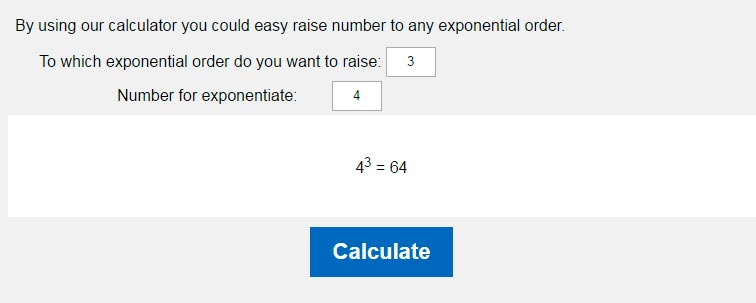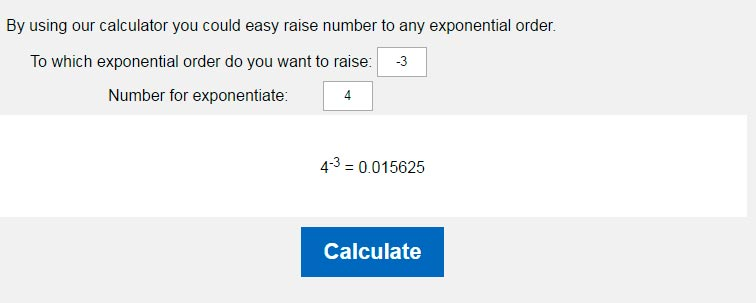# Exponents Calculator. Raising the number to exponential order.

By using our calculator you could easy raise number to any exponential order.
 To which exponential order do you want to raise: Number for exponentiate:

What is it - to raise number to exponential order? For it will be clear let's examine simple example:

43

where 4 - base, 3 – exponential order for raising the base.
Solution for this sum will be the next

4*4*4 = 64.

Thereby we could perform simple summary: raising the number to exponential order is a multiplication of this number by so many times as the exponential order is.

You could raise not only to positive exponential order but to negative one too. Many people set oneself one question - how to raise the number to negative exponential order. When you raise number to negative exponential order you have to as usual raise number to exponential order and then divide it into one.
This way expression like

4-3

will be presented next manner:

1/(4*4*4)= 1/64 = 0,015625

### Raising the number to exponential order### Raising the number to negative exponential orderHOME
RESOURCES